# Solution June 29, 2007

### Problem 1

Show that the rational number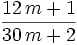${{12\,m+1}\over{30\,m+2}}$

is in lowest terms for any positive integer m.

### Solution 1

We have to show that the greatest common divisor (http://en.wikipedia.org/wiki/Greatest_common_divisor) of the numerator and denominator is 1. We can do this as follows: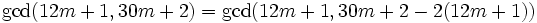$\gcd(12m+1, 30m+2) = \gcd(12m+1, 30m+2 - 2(12m+1))\,$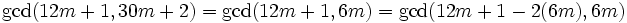$\gcd(12m+1, 30m+2) = \gcd(12m+1, 6m) = \gcd(12m+1 - 2(6m), 6m)\,$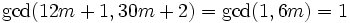$\gcd(12m+1, 30m+2) = \gcd(1, 6m) = 1\,$

q.e.d.

### Problem 2

A cube has all sides labeled with a positive integer. Then at each corner of the cube the corner is labeled with the product of the numbers on the three sides that come together at the corner. The sum of the numbers from all 8 corners is 1001. What is the sum of the 6 numbers on the sides?

### Solution 2

Let the numbers on the faces be a,b,c,d,e,f, where the pairs (a,b), (c,d) and (e,f) are the numbers on opposite sides.

Then,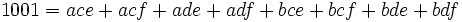$1001 = ace + acf + ade + adf + bce + bcf + bde + bdf\,$

and we can factor out (a + b) and then (c + d) to obtain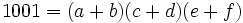$1001 = (a+b)(c+d)(e+f)\,$.

Now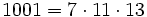$1001 = 7\cdot 11 \cdot 13$, and all these factors are prime. Because a and b are positive,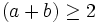$(a+b)\geq2$ and likewise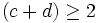$(c+d)\geq2$ and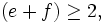$(e+f)\geq2,$ so each of these factors contains at least one prime factor. As there are only 3 prime factors available, these three sums must be 7, 11 and 13 in some order.

But addition is commutative. Therefore,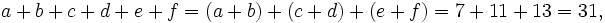$a+b+c+d+e+f = (a+b)+(c+d)+(e+f) = 7+11+13 = 31,\,$

so the sum of the numbers on the sides is 31.